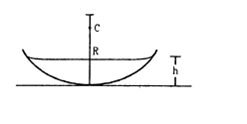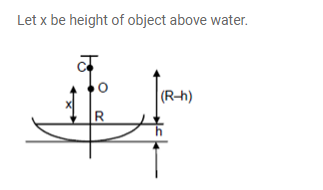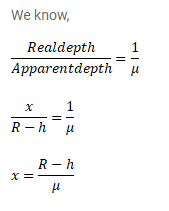# A concave mirror of radius`
Question:

A concave mirror of radius $\mathrm{R}$ is kept on a horizontal table. Water (refractive index $=\mu$ ) is poured into it up to a height $h$. Where an object should be placed so that its image is formed on itself?Solution: